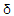# Electronics - Field Effect Transistors (FET)

### Exercise :: Field Effect Transistors (FET) - Filling the Blanks

1.

To get a negative gate-source voltage in a self-biased JFET circuit, you must use a ________.

 A. negative gate supply voltage B. ground C. voltage divider D. source resistor

Answer: Option D

Explanation:

No answer description available for this question. Let us discuss.

2.

One advantage of voltage-divider bias is that the dependency of drain current, ID, on the range of Q points is _________.

 A. reduced B. increased C. not affected D. none of the above

Answer: Option A

Explanation:

No answer description available for this question. Let us discuss.

3.

Changing ________ can control the voltage gain of a common-source amplifier.

 A. the input voltage B.m C. VDD D. RS

Answer: Option B

Explanation:

No answer description available for this question. Let us discuss.

4.

A __________ change in VDS will produce a __________ change in ID.

 A. small, large B. large, small C. large, large D. small, small

Answer: Option B

Explanation:

No answer description available for this question. Let us discuss.

5.

A _______________ JFET amplifier provides a voltage gain of less than one.

 A. common-source B. common-gate C. common-drain D. cascode amplifier

Answer: Option C

Explanation:

No answer description available for this question. Let us discuss.

#### Current Affairs 2021

Interview Questions and Answers